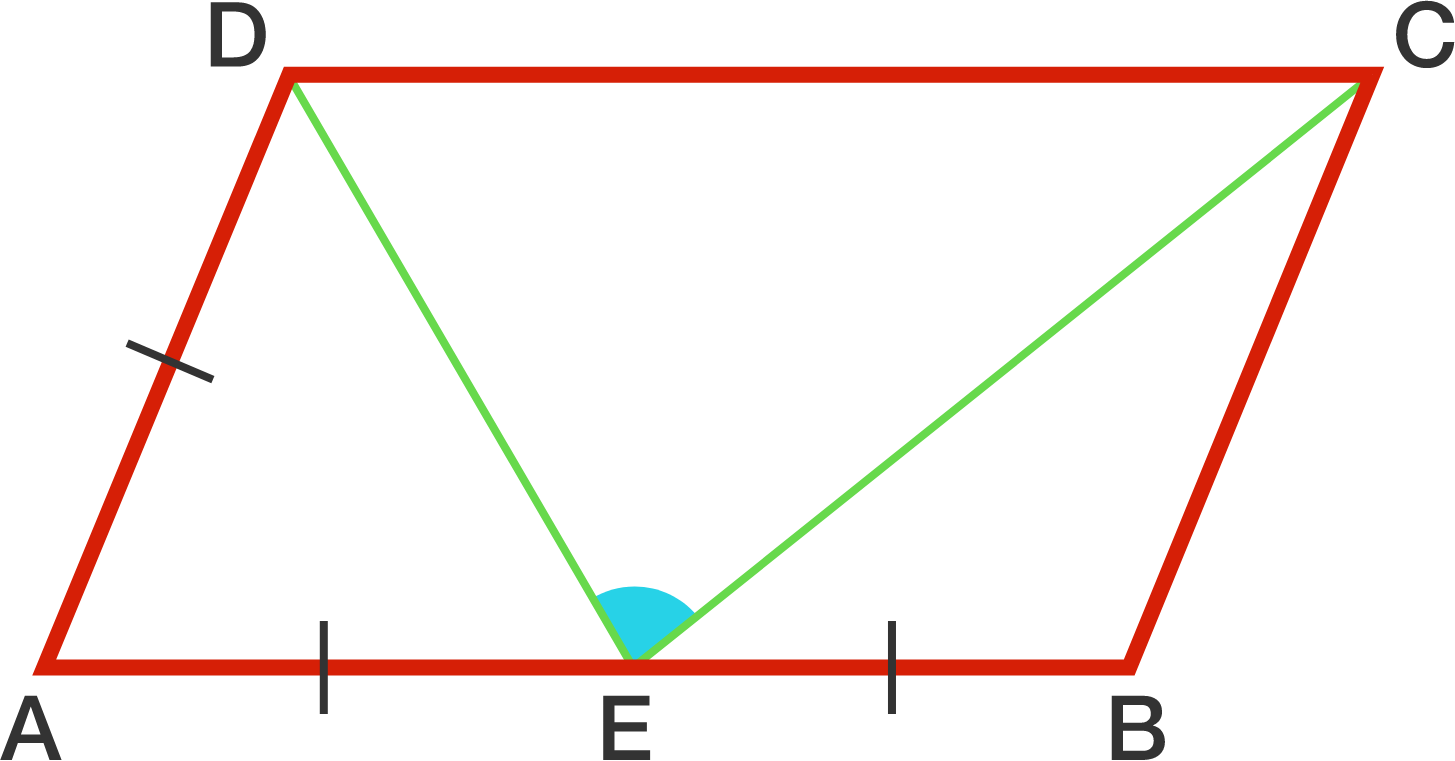# ParallelogramaniaIn a parallelogram $ABCD$, the length of $AB$ is twice the length of $AD$. $E$ is the midpoint of $AB$.

Find the measure of $\angle DEC$ in degrees.

×